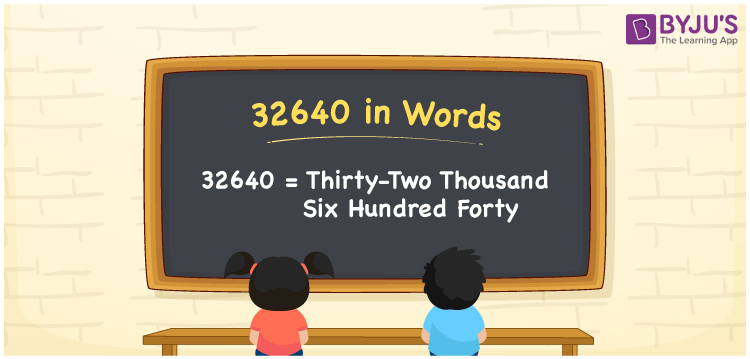# 32640 in Words

32640 in words is Thirty-two thousand six hundred forty. If you earned Rs. 32640 in a month, it can be written as “I earned Rs. Thirty-two thousand six hundred forty in a month”. As we know, 32640 is a cardinal number and its number name can be written using the place value system. The number 32640 is a cardinal number, as it is used to count things, measure distance, relate to money and so on.

 32640 in Words: Thirty-two Thousand Six Hundred Forty. Thirty-two Thousand Six Hundred Forty in Numerical Form: 32640.

## 32640 in English Words## How to Write 32640 in Words?

The following table depicts the place values of the number 32640:

 Ten-thousands Thousands Hundreds Tens Ones 3 2 6 4 0

The expanded form of 32640 is as follows:

= 3 × Ten thousand + 2 × Thousand + 6 × Hundred + 4 × Ten + 0 × One

= 3 × 10000 + 2 × 1000 + 6 × 100 + 4 × 10 + 0 × 1

= 30000 + 2000 + 600 + 40

= 32640

= Thirty-two thousand six hundred forty

Hence, 32640 in words is thirty-two thousand six hundred forty.

32640 in words – Thirty-two thousand six hundred forty

Is 32640 an odd number? – No

Is 32640 an even number? – Yes

Is 32640 a perfect square number? – No

Is 32640 a perfect cube number? – No

Is 32640 a prime number? – No

Is 32640 a composite number? – Yes

## Frequently Asked Questions on 32640 in Words

Q1

### Write 32640 in words.

32640 in words is thirty-two thousand six hundred forty.

Q2

### Simplify 32000 + 640, and express it in words.

Simplifying 32000 + 640, we get 32640. Hence, 32640 in words is thirty-two thousand six hundred forty.

Q3

### How to write thirty-two thousand six hundred forty in numbers?

Thirty-two thousand six hundred forty in numbers is 32640.